Unlimited PowerPoint templates, graphics, videos & courses! Unlimited asset downloads! From $16.50/m Advertisement# How to Link Your Data in Excel Workbooks Together Read Time:8 minsLanguages: As you use and build more Excel workbooks, you'll need to link them up. Maybe you want to write formulas that use data between different sheets in a workbook. You can even write formulas that use data from multiple different workbooks. If I want to keep my files clean and tidy, I've found it's best to separate large sheets of data from the formulas that summarize them. I often use a single workbook or sheet to summarize things. In this tutorial, you'll learn how to link data in Excel. First, we'll learn how to link up data in the same workbook on different sheets. Then, we'll move on to linking up multiple Excel workbooks to import and sync data between files. ## How to Quickly Link Data in Excel Workbooks (Watch & Learn) I'll walk you through two examples linking up your spreadsheets. You'll see how to pull data from another workbook in Excel and keep two workbooks connected. We'll also walk through a basic example to write formulas between sheets in the same workbook. Let's walk through an illustrated guide to linking up your data between sheets and workbooks in Excel. ## Basics: How to Link Between Sheets in Excel Let's start off by learning how to write formulas using data from another sheet. You probably already know that Excel workbooks can contain multiple worksheets. Each worksheet is a tab of its own, and you can switch tabs by clicking on them at the bottom of Excel. Complex workbooks can easily grow to many sheets. In time, you'll certainly need to write formulas to work with data on different tabs. Maybe you use a single sheet in your workbook for all of your formulas to summarize your data, and separate sheets to hold the original data.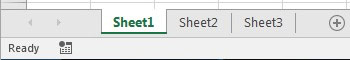My spreadsheet has three tabs on it. I'll write a formula to work with data from each sheet. Let's learn how to write a multi-sheet formula to work with data from multiple sheets in the same workbook. ### 1. Start a New Formula in Excel Most formulas in Excel start off with the equals (=) sign. Double click or start typing in a cell and begin writing the formula that you want to link up. For my example, I'll write a sum formula to add up several cells. I'll open up the sign, and then click on the first cell on my current sheet to make it the first part of the formula. Then, I'll type a sign to add my second cell to this formula.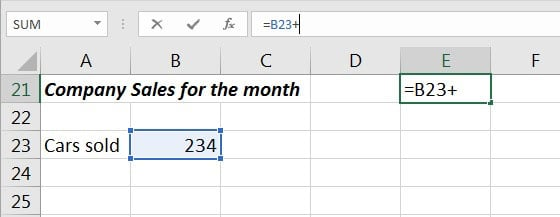Start writing a formula in a cell and click on the first cell reference to include, but make sure not to close out the formula yet. Now, make sure that you don't close out your formula and press enter yet! You'll want to leave the formula open before you switch sheets. ### 2. Switch Sheets in Excel While you still have the formula open, click on a different sheet tab at the bottom of Excel. It's very important that you don't close out the formula before you click on the next cell to include as part of the formula.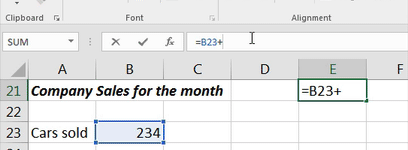Jump to different sheet in Excel. After you switch sheets, click on the next cell that you want to include in the formula. As you can see in the screenshot below, Excel automatically writes the part of the formula that references a cell on another sheet for you. Notice in the screenshot below that to reference a cell on another sheet, Excel adds "Sheet2!B3", which simply references cell B3 on a sheet named Sheet2. You could write this manually, but clicking on the cells makes Excel write it for you automatically.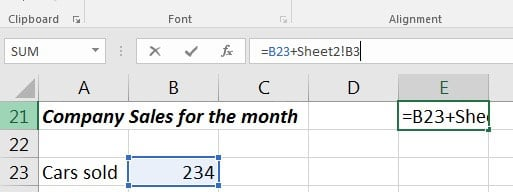Excel automatically writes part of the formula for you to reference a cell on another sheet. ### 3. Finish the Excel Formula At this point, you can press enter to close out and complete your multi-sheet formula. When you do so, Excel will jump back to where you started the formula and show you the results. You could also keep writing the formula, including cells from more sheets and other cells on the same sheet. Keep combining those references throughout the workbook for all the data you need. ## Level Up: How to Link Multiple Excel Workbooks Let's learn how to pull data from another workbook. With this skill, you can write formulas that pull together data from entirely separate Excel workbooks. For this section of the tutorial, you can use two workbooks that you can download for free as a part of this tutorial. Open them both up in Excel, and follow the directions below. ### 1. Open Both Workbooks Let's start off by writing a formula that includes data from two different workbooks. The easiest way to use this feature is to open up two Excel workbooks at the same time and put them side by side. I use the Windows Snap feature to split them to each take up half the screen. You need to keep both workbooks in view to write formulas between them. In the screenshot below, I've opened two workbooks that I'll write formulas for side-by-side. For my example, I'm running a business that buys a variety of products, and sells them in a variety of countries. So, I'll use separate workbooks to track my purchases/sales and cost data.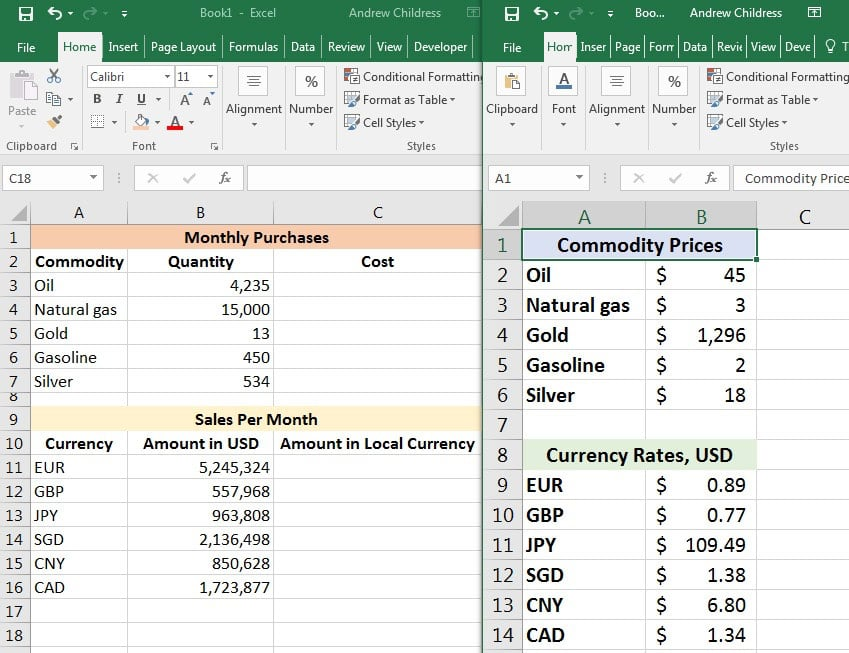For this example, I'm using separate workbooks to track my purchases/sales and cost data. ### 2. Start Writing Your Formula in Excel The price of what I buy can change, and so can the rate that I receive payments in. I need to keep a lookup list of rates and multiply it times my purchases. This is the perfect time to link two workbooks together and write formulas between them. Let's take the number of barrels of oil I buy each month times the price per barrel. In the first Cost cell (cell C3), I'll start writing a formula by typing the equals sign (=), and then clicking on cell B3 to grab the quantity. Now, I'll add an * to prepare to multiply the quantity by the rate. So far, your formula should be: =B3* Don't close out your formula yet. Make sure to leave it open before moving onto the next step; we still need to point Excel to the price data to multiply the quantity by. ### 3. Switch Excel Workbooks It's time to switch workbooks, and this is why it's important to keep both of your datasets in view while working between workbooks. With your formula still open, click over to the other workbook. Then, click on a cell in your second workbook to link up the two Excel files. Excel automatically wrote the reference to a separate workbook as part of the cell formula: =B3*[Prices.xlsx]Sheet1!$B$2 Once you press Enter, Excel will calculate the final cost by multiplying the quantity in the first workbook times the price in the second workbook. Now, keep working on your Excel skills by multiplying each of the quantities or values times the reference amounts in the "Prices" workbook. In short, the key is to get your workbooks open side by side, and simply switch workbooks to write formulas referencing other files. There's nothing stopping you from linking up more than two workbooks. You could open many workbooks to link up and write formulas, connecting the data between many sheets to keep cells up to date. ## How to Refresh Your Data Between Workbooks When you've written formulas that reference other Excel workbooks, you'll need to think about how you'll update your data. So, what happens when the data changes in the workbook that you're linking to? Will your workbook automatically update, or will you need to refresh your files to pull over the last data and import it? The answer is, "it depends", and specifically, it depends upon if both workbooks are still open at the same time. ### Example 1: Both Excel Workbooks Still Open Let's check out an example using the same workbook from the prior step. Both workbooks are still open. Let's see what happens when we change the price of oil from$45 per barrel to \$75 per barrel:

In the screenshot above, you can see that when we updated the price of oil, the other workbook automatically updated.

This is important to know: if both workbooks are open at the same time, changes will update automatically and in real-time. When you change one variable, the other workbook will update or recalculate based upon the new value.

### Example 2: With One Workbook Closed

What if you only open one workbook at a time? For example, each morning, we update prices of our commodities and currencies, and in the evening we review the impact of the change to our purchases and sales.

The next time you open up your workbook that references other sheets, you might get a message similar to the one below. You can click on Update to pull in the latest data from your reference workbook.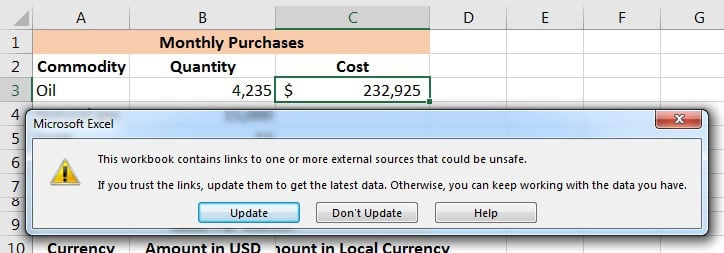Click update on the pop-up that shows when opening the workbook to pull the latest values from the separate file.

You might also see a menu where you can click Enable Content to automate updating data between Excel files.

## Recap and Keep Learning More About Excel

Writing formulas between sheets and workbooks is a necessary skill when you work with Microsoft Excel. Using multiple spreadsheets inside your formulas is no problem with a bit of know-how.

Check out these additional tutorials to learn more about Excel skills and how to work with data. These tutorials are a great way to continue learning Excel.